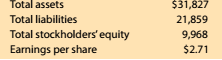Chapter 8, Problem 8.9.1MBA

Chapter
Section
Textbook Problem

Debt and price-earnings ratios Lowe's Companies Inc. (LOW) operates over 1,800 home improvement retail stores and is a competitor of The Home Depot (HD). The following data (in millions) were adapted from a recent financial statement of Lowe's:Compute the debt ratio. Round lo one decimal place.

To determine

Concept Introduction:

Debt Ratio:

Debt ratio is the relationship between the Total liabilities and Total Assets of a corporation. Debt ratio shows the part of assets financed by debts. It is calculated by dividing total liabilities by total assets. The formula of debt ratio is as follows:

Debt Ratio = Total LiabilitiesTotal Assets

To Calculate:

The Debt Ratios

Explanation

The Debt ratio is calculated as follows:

 \$ in Millions Total Liabilities (A)

Still sussing out bartleby?

Check out a sample textbook solution.

See a sample solution

The Solution to Your Study Problems

Bartleby provides explanations to thousands of textbook problems written by our experts, many with advanced degrees!

Get Started

PRESENT AND FUTURE VALUES Of A CASH FLOW STREAM An investment will pay 100 at the end of each of the next 3 sta...

Fundamentals of Financial Management, Concise Edition (with Thomson ONE - Business School Edition, 1 term (6 months) Printed Access Card) (MindTap Course List)

What are the advantages of a corporation?

Foundations of Business (MindTap Course List)

What is a separation report?

PAYROLL ACCT.,2019 ED.(LL)-TEXT